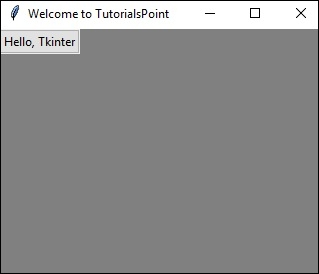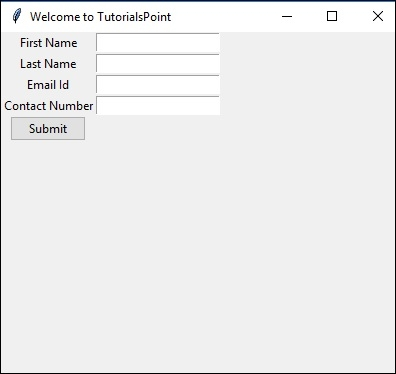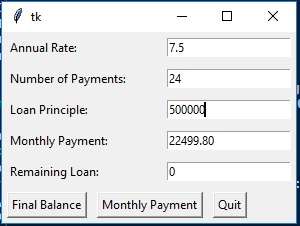# Simple registration form using Python Tkinter

Tkinter is a python library for developing GUI (Graphical User Interfaces). We use the tkinter library for creating an application of UI (User Interface), to create windows and all other graphical user interfaces.

If you’re using python 3.x(which is recommended), Tkinter will come with Python as a standard package, so we don’t need to install anything to use it.

Before creating a registration form in Tkinter, let’s first create a simple GUI application in Tkinter.

## Creating a simple GUI application

Below is the program to create a window by just importing Tkinter and set its title −

from tkinter import *
from tkinter import ttk
window = Tk()
window.title("Welcome to TutorialsPoint")
window.geometry('325x250')
window.configure(background = "gray")
ttk.Button(window, text="Hello, Tkinter").grid()
window.mainloop()

On running above lines of code, you will see the output something like −Let's understand the above lines of code −

• Firstly we import all the modules we need, we have imported ttk and * (all) from tkinter library.

• To create the main window of our application, we use Tk class.

• window.title(), give the title to our Window app.

• window.geometry(), set the size of the window and window.configure(), set its background color.

• ttk.Button() makes a button.

• ttk.Button(window, text="Hello, Tkinter").grid() – window means Tk so it shows in the window we created, text- will display the text in the window and grid will make it in a grid.

• Window.mainloop(), this function calls the endless loop of the window, so will remain open till the user closes it.

Let’s try to extend our previous example, by adding a couple of Labels (label is a simple widget which displays a piece of text or image) and button(button are usually mapped directly onto a user action which means on clicking a button, some action should occur) in the code.

from tkinter import *
from tkinter import ttk
window = Tk()
window.title("Welcome to TutorialsPoint")
window.geometry('400x400')
window.configure(background = "grey");
a = Label(window ,text = "First Name").grid(row = 0,column = 0)
b = Label(window ,text = "Last Name").grid(row = 1,column = 0)
c = Label(window ,text = "Email Id").grid(row = 2,column = 0)
d = Label(window ,text = "Contact Number").grid(row = 3,column = 0)
a1 = Entry(window).grid(row = 0,column = 1)
b1 = Entry(window).grid(row = 1,column = 1)
c1 = Entry(window).grid(row = 2,column = 1)
d1 = Entry(window).grid(row = 3,column = 1)
def clicked():
res = "Welcome to " + txt.get()
lbl.configure(text= res)
btn = ttk.Button(window ,text="Submit").grid(row=4,column=0)
window.mainloop()

On running the above code, we will see the output screen something like −Now let’s create something from the real world, maybe a loan interest calculator. For that, we need a couple of items(variables) to be known, like principal amount, loan rate (r), balance (Bs) after s payments. To calculate loan after s payment, we use the formula in below program −

Ps = ((1+r)^s.Bo) – (((1 + r)^s – 1)/ r)*p

Where −

Rate = Rate of interest like 7.5%

i = rate/100, annual rate in decimal

r = period rate = i/12

Po = Principal amount

Ps = Balance after s payments

s = number of monthly payments

p = period (monthly) payment

So below is the Interest rate calculator program, which will show a pop-up window, where users can set desired value (loan amount, rate, number of installments) and will get the monthly payment amount and remaining loan he needs to pay with the help of python tkinter library.

from tkinter import *
fields = ('Annual Rate', 'Number of Payments', 'Loan Principle', 'Monthly Payment', 'Remaining Loan')
def monthly_payment(entries):
# period rate:
r = (float(entries['Annual Rate'].get()) / 100) / 12
print("r", r)
# principal loan:
loan = float(entries['Loan Principle'].get())
n = float(entries['Number of Payments'].get())
remaining_loan = float(entries['Remaining Loan'].get())
q = (1 + r)** n
monthly = r * ( (q * loan - remaining_loan) / ( q - 1 ))
monthly = ("%8.2f" % monthly).strip()
entries['Monthly Payment'].delete(0,END)
entries['Monthly Payment'].insert(0, monthly )
print("Monthly Payment: %f" % float(monthly))
def final_balance(entries):
# period rate:
r = (float(entries['Annual Rate'].get()) / 100) / 12
print("r", r)
# principal loan:
loan = float(entries['Loan Principle'].get())
n = float(entries['Number of Payments'].get())
q = (1 + r)** n
monthly = float(entries['Monthly Payment'].get())
q = (1 + r)** n
remaining = q * loan - ( (q - 1) / r) * monthly
remaining = ("%8.2f" % remaining).strip()
entries['Remaining Loan'].delete(0,END)
entries['Remaining Loan'].insert(0, remaining )
print("Remaining Loan: %f" % float(remaining))
def makeform(root, fields):
entries = {}
for field in fields:
row = Frame(root)
lab = Label(row, width=22, text=field+": ", anchor='w')
ent = Entry(row)
ent.insert(0,"0")
row.pack(side = TOP, fill = X, padx = 5 , pady = 5)
lab.pack(side = LEFT)
ent.pack(side = RIGHT, expand = YES, fill = X)
entries[field] = ent
return entries
if __name__ == '__main__':
root = Tk()
ents = makeform(root, fields)
root.bind('<Return>', (lambda event, e = ents: fetch(e)))
b1 = Button(root, text = 'Final Balance',
command=(lambda e = ents: final_balance(e)))
b2 = Button(root, text='Monthly Payment',
command=(lambda e = ents: monthly_payment(e)))
root.mainloop()